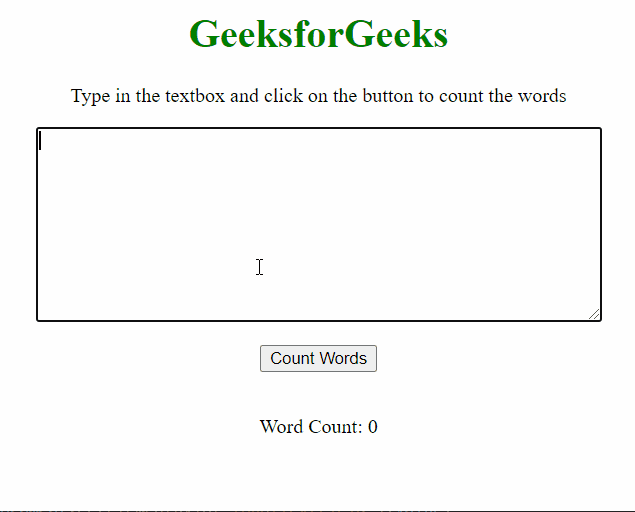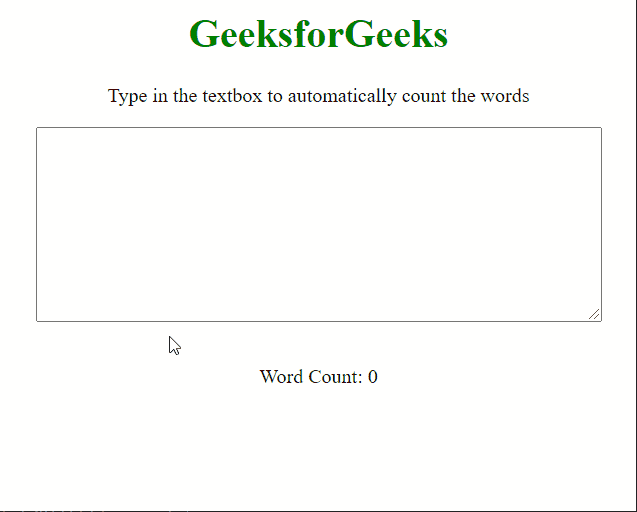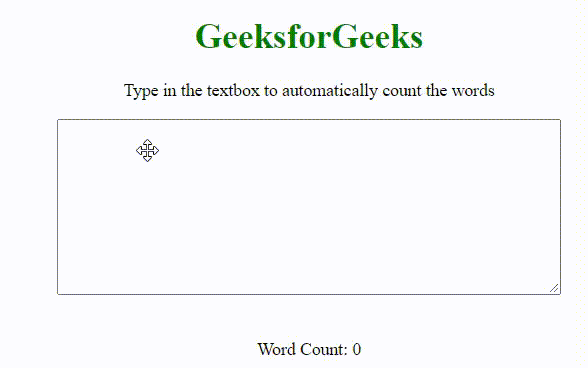Open In App

# How to make a word count in textarea using JavaScript ?

This article describes the approach to calculate the number of words in a given text input. This may be useful in scenarios where the user is recommended to enter a certain number of words and the word counter can keep track of the same. There are two approaches that are discussed below:

Approach 1: Calculating the number of spaces present in the text.

This method relies on the number of spaces present in the input string to count the number of words as every word in a sentence is separated by a space. The function countWord() is defined which takes the text present in the textarea and counts the number of spaces present in it. The input text in the textarea is selected by using the getElementById() method.

The drawback of this method is that multiple spaces between words would be counted as multiple words, hence it may cause the word count to be unreliable.

Example: This example shows the above-explained approach.

## HTML

 `<``body` `style``=``"text-align: center;"``>``    ``<``h1` `style``=``"color: green"``>``        ``GeeksforGeeks``    ```` ` `    ``<``p``>``        ``Type in the textbox and click on``        ``the button to count the words``    ```` ` `    ``<``textarea` `id``=``"inputField"` `rows``=``10` `cols``=``"60"``>``    ````    ``<``br``><``br``>`` ` `    ``<``button` `onclick``=``"countWords()"``>``        ``Count Words``    ````    ``<``br``><``br``>`` ` `    ``<``p``> Word Count:``        ``<``span` `id``=``"show"``>0``    ```` ` `    ``<``script``>``        ``function countWords() {``         ` `            ``// Get the input text value``            ``var text = document``                ``.getElementById("inputField").value;``         ` `            ``// Initialize the word counter``            ``var numWords = 0;``         ` `            ``// Loop through the text``            ``// and count spaces in it ``            ``for (var i = 0; i < ``text.length``; i++) {``                ``var ``currentCharacter` `= ``text``[i];``         ` `                ``// Check if the character is a space``                ``if (currentCharacter == " ") {``                    ``numWords += 1;``                ``}``            ``}``         ` `            ``// Add 1 to make the count equal to``            ``// the number of words ``            ``// (count of ``words` `= ``count` `of spaces + 1)``            ``numWords += 1;``         ` `            ``// Display it as output``            ``document.getElementById("show")``                ``.innerHTML` `= ``numWords``;``        ``}``    `````

Output:Approach 2: Separating the words based on spaces and then counting the number of words.

In this approach, we improve the drawback of the previous approach by splitting the words on the basis of the space character and then checking that each split is not only a space character. The countWord() function is called every time the user types in something into the text area using the oninput event handler on the text area.

Example: This example shows the above-explained approach.

## HTML

 `<``body` `style``=``"text-align: center;"``>``    ``<``h1` `style``=``"color: green"``>``        ``GeeksforGeeks``    ```` ` `    ``<``p``>``        ``Type in the textbox to``        ``automatically count the words``    ```` ` `    ``<``textarea` `id``=``"word"` `oninput``=``"countWord()"` `rows``=``"10"` `cols``=``"60"``>``    ````    ``<``br``><``br``>`` ` `    ``<``p``> Word Count:``        ``<``span` `id``=``"show"``>0``    ```` ` `    ``<``script``>``        ``function countWord() {``         ` `            ``// Get the input text value``            ``var words = document``                ``.getElementById("word").value;``         ` `            ``// Initialize the word counter``            ``var count = 0;``         ` `            ``// Split the words on each``            ``// space character ``            ``var split = words.split(' ');``         ` `            ``// Loop through the words and ``            ``// increase the counter when ``            ``// each split word is not empty``            ``for (var i = 0; i < ``split.length``; i++) {``                ``if (split[i] != "") {``                    ``count += 1;``                ``}``            ``}``         ` `            ``// Display it as output``            ``document.getElementById("show")``                ``.innerHTML` `= ``count``;``        ``}``    `````

Output:Approach 3:

Since the above two approaches were only able to count words when written in continuation giving spaces, but it was not able to count numbers when each word starts with a new line. So this approach will be able to count the words starting from the new line.

Example: This example shows the above-explained approach.

## HTML

 `<``body` `style``=``"text-align: center"``>``    ``<``h1` `style``=``"color: green"``>GeeksforGeeks`` ` `    ``<``p``>``        ``Type in the textbox to automatically count the words``        ```` ` `    ``<``textarea` `id``=``"word"` `rows``=``"10"` `cols``=``"60"``> ``    ``<``br` `/><``br` `/>`` ` `    ``<``p``>``        ``Word Count:``        ``<``span` `id``=``"show"``>0``    ```` ` `    ``<``script``>``        ``document``          ``.querySelector("#word")``          ``.addEventListener("input", function countWord() {``            ``let res = [];``            ``let str = this.value.replace(/[\t\n\r\.\?\!]/gm, " ").split(" ");``            ``str.map((s) => {``              ``let trimStr = s.trim();``              ``if (trimStr.length > 0) {``                ``res.push(trimStr);``              ``}``            ``});``            ``document.querySelector("#show").innerText = res.length;``          ``});``    `````

Output: